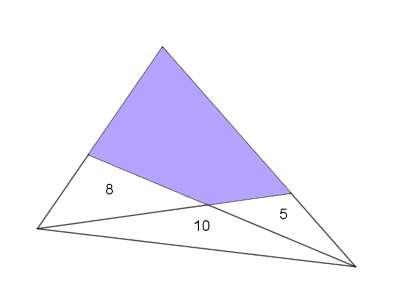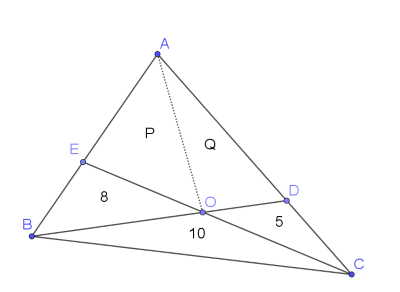#### You may also like### Napoleon's Hat

Three equilateral triangles ABC, AYX and XZB are drawn with the point X a moveable point on AB. The points P, Q and R are the centres of the three triangles. What can you say about triangle PQR?### Plane to See

P is the midpoint of an edge of a cube and Q divides another edge in the ratio 1 to 4. Find the ratio of the volumes of the two pieces of the cube cut by a plane through PQ and a vertex.### Golden Ratio

Solve an equation involving the Golden Ratio phi where the unknown occurs as a power of phi.

# Areas and Ratios

### Why do this problem?

This problem presents a geometrical situation where it appears there is not enough information to solve the problem. However, by persevering and making use of the hints given in the task, students will be surprised to discover that an elegant solution can be found.

### Possible approach

Begin by sharing this image and invite students to suggest a lower and upper bound for the shaded area."Do you think we have enough information to work out the area exactly?"
Give students some time to think about what they know and what they can work out. They may conclude that there's not enough information.

"Sometimes we can get insights from looking at a simpler problem first..."
Share the interactive GeoGebra applet from the problem and invite students to suggest and explain a relationship between the bases and the areas of the triangles. Then refer back to the original problem - "Are there any relationships between lengths that we can deduce?"

"Another useful tool for solving geometry problems is to label our diagram to make it easier to talk about, and add in extra lines..."
Show this image:"Can you find expressions which include P and Q based on what we know about areas and lengths? Does this help us to solve the problem?"

Finally, encourage students to write up their solution formally, making sure that they justify each step of the method clearly.

### Key questions

What do we know?
What can we deduce?
If $O$ lies on $BD$, and $BOC$ has twice the area of $DOC$, what can we say about the lengths $BO$ and $OD$?
What does this tell us about areas $BOA$ and $AOD$?

### Possible support

Students could start by exploring Triangle in a Triangle.

### Possible extension

Another Triangle in a Triangle provides a suitable follow-up challenge that uses similar ideas.